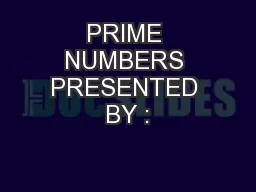# PRIME NUMBERS PRESENTED BY :2018-02-11 27K 27 0 0

## PRIME NUMBERS PRESENTED BY : - Description

NANDAN GOEL. HISTORY. THE STUDY OF SURVIVING RECORDS OF EGYPTIANS SHOW THAT THEY HAD KNOWLEDGE OF PRIMES.. THE GREEK MATHEMATICIAN . “. EUCLID PERFORMED. ”. SOME EXCEPTIONAL WORK .. HIS WORK . “. ID: 630520 Download Presentation

Embed code:

## PRIME NUMBERS PRESENTED BY :

Download Presentation - The PPT/PDF document "PRIME NUMBERS PRESENTED BY :" is the property of its rightful owner. Permission is granted to download and print the materials on this web site for personal, non-commercial use only, and to display it on your personal computer provided you do not modify the materials and that you retain all copyright notices contained in the materials. By downloading content from our website, you accept the terms of this agreement.

### Presentations text content in PRIME NUMBERS PRESENTED BY :

Slide1

PRIME NUMBERS

PRESENTED BY :

NANDAN GOEL

Slide2

HISTORY

THE STUDY OF SURVIVING RECORDS OF EGYPTIANS SHOW THAT THEY HAD KNOWLEDGE OF PRIMES.

THE GREEK MATHEMATICIAN “

EUCLID PERFORMED

SOME EXCEPTIONAL WORK .

HIS WORK

EUCLID ELEMENT

CONTAIN IMPORTANT THEOREMS SUCH AS INFINITUDE OF PRIMES AND BASIC THEOREM OF ARITHMETIC.

THE FRENCH MONK

MARIN MERSENNE

LOOKED AT PRIMES OF THE FORM 2

P

− 1, WITH

P

A PRIME. THEY ARE CALLED MERSENNE PRIMES IN HIS HONOR.

Slide3

PRIME NUMBER THEOREM

LET

Π

(

X

) BE THE PRIME – COUNTING FUNCTION THAT GIVES THE NUMBER OF PRIMES LESS THAN OR EQUAL TO

X

, FOR ANY REAL NUMBER

X. FOR EXAMPLE, Π(10) = 4 BECAUSE THERE ARE FOUR PRIME NUMBERS (2, 3, 5 AND 7) LESS THAN OR EQUAL TO 10. THE PRIME NUMBER THEOREM THEN STATES THAT X / LOG X IS A GOOD APPROXIMATION TO Π(X), IN THE SENSE THAT THE LIMIT OF THE QUOTIENT OF THE TWO FUNCTIONS Π(X) AND X / LOG X AS X INCREASES WITHOUT BOUND IS 1.

Slide4

TESTING PRIMALITY

THE MOST BASIC METHOD OF CHECKING THE PRIMALITY OF A GIVEN INTEGER

N IS CALLED

TRIAL

DIVISION.

THIS ROUTINE CONSISTS OF DIVIDING

N

BY EACH INTEGER

M THAT IS GREATER THAN 1 AND LESS THAN OR EQUAL TO THE SQUARE ROOT OF N.IF THE RESULT OF ANY OF THESE DIVISIONS IS AN INTEGER, THEN N IS NOT A PRIME, OTHERWISE IT IS A PRIME.INDEED, IF N = ab IS COMPOSITE (WITH a AND b ≠ 1) THEN ONE OF THE FACTORS a OR b IS NECESSARILY AT MOST √N.

Slide5

SIEVE OF ERATOSTHENES

Slide6

MODERN PRIMALITY TESTS

AKS primality test

2002

deterministic

O(log

6+

ε

(

n))Baillie PSW primality test1980probabilisticO(log3 n

)

no known counterexamples

Elliptic curve primality proving

1977

deterministic

O(log

5+

ε

(

n

))

heuristically

Fermat primality

t

est

probabilistic

O(

k

· log

2+

ε

(

n

))

fails for Carmichael numbers

Miller

Rabin primality

test

1980

probabilistic

O(

k

· log

2+

ε

(

n

))

error probability 4

k

Solovay

S

trassen primalit

y

test

1977

probabilistic

O(

k

· log

3

n

)

error probability 2

k

Slide7

FERMAT PRIMALITY TEST

FERMAT

‘S THEOREM STATES THAT

N

P

≡N (MOD P)

FOR

ANY N IF P IS A PRIME. IF WE HAVE A NUMBER B THAT WE WANT TO TEST FOR PRIMALITY.THEN WE WORK OUT NB (MOD B) FOR A RANDOM VALUE OF N AS OUR TEST. A FLAW WITH THIS TEST IS THAT THERE ARE SOME COMPOSITE NUMBERS THAT SATISFY THE FERMAT IDENTITY EVEN THOUGH THEY ARE NOT PRIME.SINCE THESE COMPOSITE NUMBERS ARE VERY RARE AS COMPARED TO PRIMES SO THIS TEST CAN BE USEFUL FOR PRACTICAL PURPOSES.

Slide8

PRIMES IN NATURE

Slide9

PRIMES IN ART

OLIVIER MESSIAEN

COMMUNICATION WITH ALIENS

Slide10

PRIMES:APPLICATION

PUBLIC KEY CRYPTOGRAPHYHASH TABLESLARGE NUMBER GENERATORS

Slide11

THANK YOU.

ANY QUERIES?